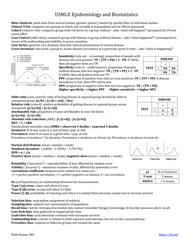2.8K
Description

```USMLE Epidemiology and Biostatistics Summary

Meta-Analysis: pools data from several studies (greater power), limited by quality/bias of individual studies

Clinical Trial: compares two groups in which one variable is manipulated and its effects measured

Cohort (relative risk): compares group with risk factor to a group without – asks “what will happen?” (prospective). Proves

cause-effect

Case Control (odds ratio): compares group with disease to group without disease – asks “what happened?” (retrospective).

Issues with confounding and inability to prove causation

Case Series: good for rare diseases, describe clinical presentation of certain disease

Cross-Sectional: data from a group to assess disease prevalence at a particular point in time – asks “what is happening?”

Sensitivity (rule out – screening): proportion of people with

disease who test positive: TP / (TP + FN) = 1 - FN. If 100%,

then all negative tests are TN.

Specificity (rule in – confirmatory): proportion of people

without disease who test negative: TN / (TN + FP) = 1 - FP.

If 100%, then all positive tests are TP.

PPV: proportion of positive tests that are true positives: TP / (TP + FP). If disease

prevalence is low, then PPV will be low.

NPV: proportion of negative tests that are true negatives. TN / (TN + FN)

Higher specificity -> higher PPV       Higher sensitivity -> higher NPV

Odds ratio (case control): odds of having disease in exposed group divided by odds in

unexposed group. (a/b) / (c/d) = (ad) / (bc)

Relative risk (cohort): relative probability of getting disease in exposed group versus

unexposed. [a/(a+b)] / [c/(c+d)]

Attributable risk: proportion of cases attributable to one risk factor.

[a/(a+b)] - [c/(c+d)]

Absolute risk reduction (ARR):  [c/(c+d)] - [a/(a+b)]

NNT = 1 / ARR

Standardized mortality ratio (SMR) = observed No deaths / expected No deaths

Incidence: No of new cases in a unit of time/ pop. at risk

Prevalence: total No of cases at a given time / pop. at risk

Prevalence = incidence * dz duration. Prevalence > incidence in chronic dz. Prevalence = incidence in acute dz

Normal distribution: mean = median = mode

Standard deviation: 1 (68%) – 2 (95%) – 3 (99.7%)

SEM = σ / √n

Positive skew (mean > median > mode), negative skew (mean < median < mode)

Reliability (“precision”) – reproducibility of test. Affected by random error

Validity (“accuracy”) – measures trueness of data. Affected by systematic error

Correlation coefficient measures how related two values are:

+1 = perfect positive correlation, -1 = perfect negative correlation, 0 = no correlation

H0 (null hypothesis): no relationship between two measurements

Type I (α) error: reject null when it’s true

Type II (β) error: accept null when it’s false

Power (1-β): probability of rejecting null when it is indeed false (increase sample size to increase power)

Selection bias: nonrandom assignment of subjects

Sampling bias: subjects not representative of population

Recall bias: risk for retrospective studies (pts cannot remember things); knowledge of disorder presence alters recall

Late-look bias: data gathered at inappropriate time

Lead-time bias: early detection confused with increased survival

Confounding bias: a factor is related to both exposure and outcome, but not on the causal pathway

Procedure bias: subjects in different groups not treated the same

- Rishi Kumar, MD @rishimd

#USMLE #Epidemiology #Biostatistics #formulas #calculations #diagnosis```
Medical jobs
view all